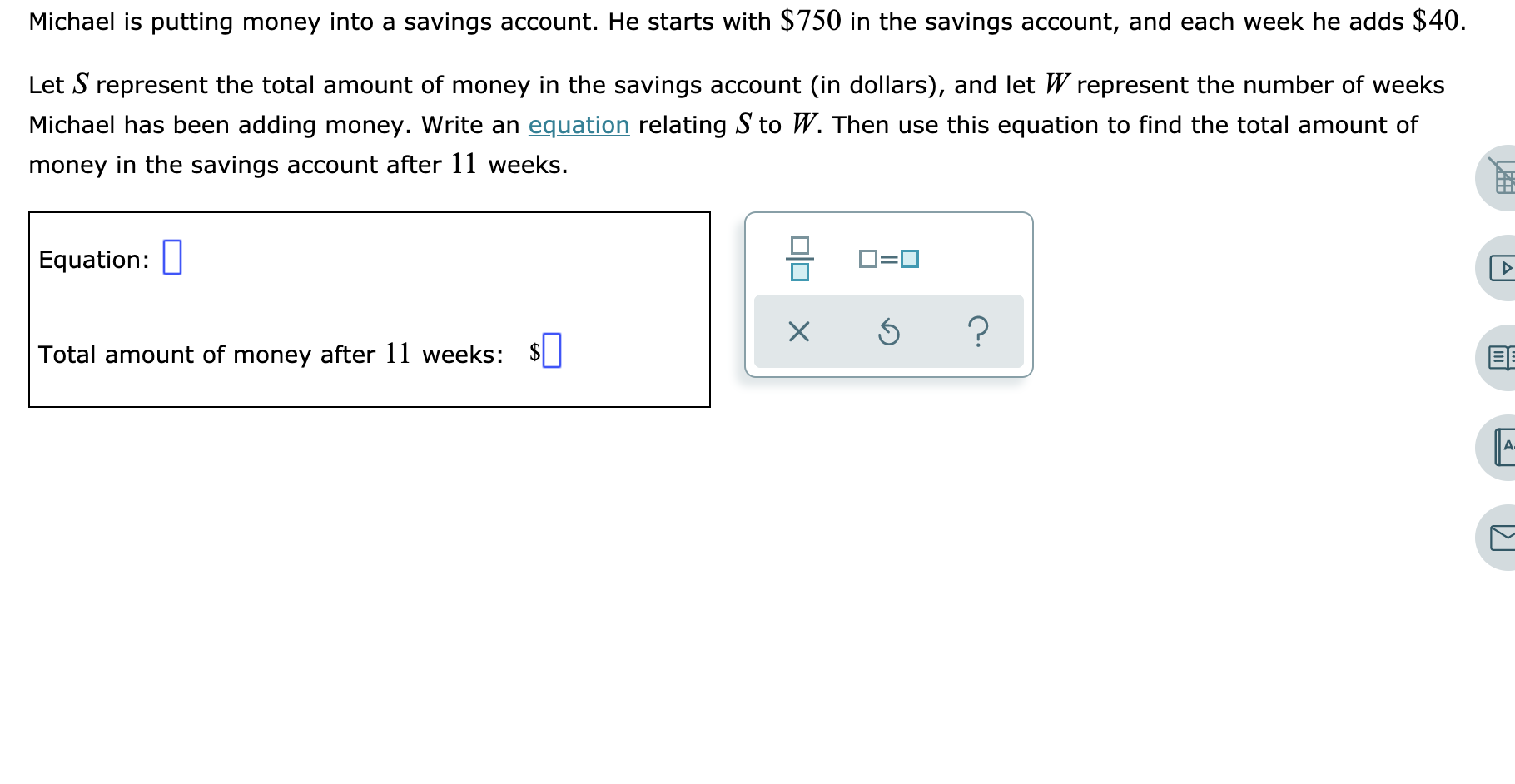# Michael is putting money into a savings account. He starts with \$750 in the savings account, and each week he adds \$40Let S represent the total amount of money in the savings account (in dollars), and let W represent the number of weeksMichael has been adding money. Write an equation relating S to W. Then use this equation to find the total amount ofmoney in the savings account after 11 weeks.Equation:?XTotal amount of money after 11 weeks:A

Question
3 views

See attachedhelp_outlineImage TranscriptioncloseMichael is putting money into a savings account. He starts with \$750 in the savings account, and each week he adds \$40 Let S represent the total amount of money in the savings account (in dollars), and let W represent the number of weeks Michael has been adding money. Write an equation relating S to W. Then use this equation to find the total amount of money in the savings account after 11 weeks. Equation: ? X Total amount of money after 11 weeks: A fullscreen
check_circle

Step 1

Let S (in dollars) represents total amount of money in saving account after W weeks. Since he adds \$40 every week, so after W weeks  the added amount is 40W.

Step 2

Total amount of money after 11 weeks can be fou...

### Want to see the full answer?

See Solution

#### Want to see this answer and more?

Solutions are written by subject experts who are available 24/7. Questions are typically answered within 1 hour.*

See Solution
*Response times may vary by subject and question.
Tagged in
MathCalculus

### Other